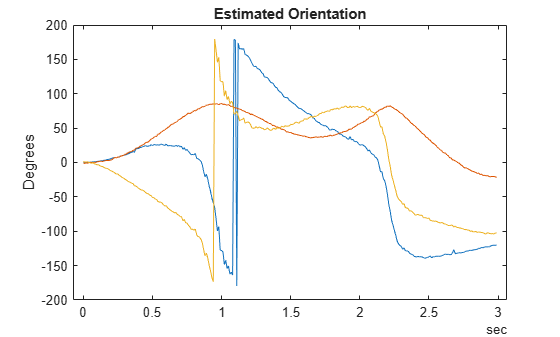# estimateStates

Batch fusion of sensor data

## Syntax

``estimates = estimateStates(filter,sensorData,measurementNoise)``

## Description

example

````estimates = estimateStates(filter,sensorData,measurementNoise)` returns the state estimates based on the motion model used in the filter, the sensor data, and the measurement noise. The function predicts the filter state estimates forward in time based on the row times in `sensorData` and fuses data from each column of the table one by one. ```

## Examples

collapse all

Load measurement data from an accelerometer and a gyroscope.

`load("accelGyroINSEKFData.mat");`

Create an `insEKF` filter object. Specify the orientation part of the state in the filter using the initial orientation from the measurement data. Specify the diagonal elements of the state estimate error covariance matrix corresponding to the orientation state as `0.01`.

```filt = insEKF; stateparts(filt,"Orientation",compact(ld.initOrient)); statecovparts(filt,"Orientation",1e-2);```

Specify the measurement noise and the additive process noise. You can obtain these values by using the `tune` object function of the filter object.

```measureNoise = struct("AccelerometerNoise", 0.1739, ... "GyroscopeNoise", 1.1129); processNoise = diag([ ... 2.8586 1.3718 0.8956 3.2148 4.3574 2.5411 3.2148 0.5465 0.2811 ... 1.7149 0.1739 0.7752 0.1739]); filt.AdditiveProcessNoise = processNoise;```

Batch-estimate the states using the `estimateStates` object function.

`estimates = estimateStates(filt,ld.sensorData,measureNoise);`

Visualize the estimate error, in quaternion distance, using the `dist` object function of the `quaternion` object.

```figure plot(rad2deg(dist(estimates.Orientation,ld.groundTruth.Orientation))) xlabel("Samples") ylabel("Distance (degrees)") title("Orientation Estimate Error")```## Input Arguments

collapse all

INS filter, specified as an `insEKF` object.

Sensor data, specified as a `timetable`. Each variable name (as a column) in the timetable must match one of the sensor names specified in the `SensorNames` property of the `filter`. Each entry in the table is the measurement from the sensor at the corresponding row time.

If a sensor does not produce measurements at a row time, specify the corresponding entry as `NaN`.

Measurement noise of the sensors, specified as a structure. Each field name must match one of the sensor names specified in the `SensorNames` property of the `filter`. The field value is the corresponding measurement noise covariance matrix. If you specify a field value as a scalar, the function extends the scalar to the diagonal of the matrix.

Data Types: `struct`

## Output Arguments

collapse all

State estimates, returned as a `timetable`. Each variable name of the table is a state name that you can obtain using the `stateinfo` object function of the filter. The last column of the table is the state estimate error covariance matrix for the complete state vector of the filter at each of the row times.

## Version History

Introduced in R2022a